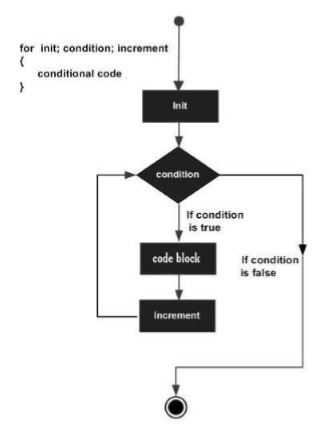# Groovy - For Statement

The for statement is used to iterate through a set of values. The for statement is generally used in the following way.

```for(variable declaration;expression;Increment) {
statement #1
statement #2
…
}
```

The classic for statement consists of the following parts −

• Variable declaration − This step is executed only once for the entire loop and used to declare any variables which will be used within the loop.

• Expression − This will consists of an expression which will be evaluated for each iteration of the loop.

• The increment section will contain the logic needed increment the variable declared in the for statement.

The following diagram shows the diagrammatic explanation of this loop.Following is an example of the classic for statement −

```class Example {
static void main(String[] args) {

for(int i = 0;i<5;i++) {
println(i);
}

}
}
```

In the above example, we are in our for loop doing three things −

• Declaring a variable i and Initializing the value of i to 0

• Putting a conditional expression that the for loop should execute till the value of i is less than 5.

• Increment the value of i by 1 for each iteration.

The output of the above code would be −

```0
1
2
3
4
```
groovy_loops.htm